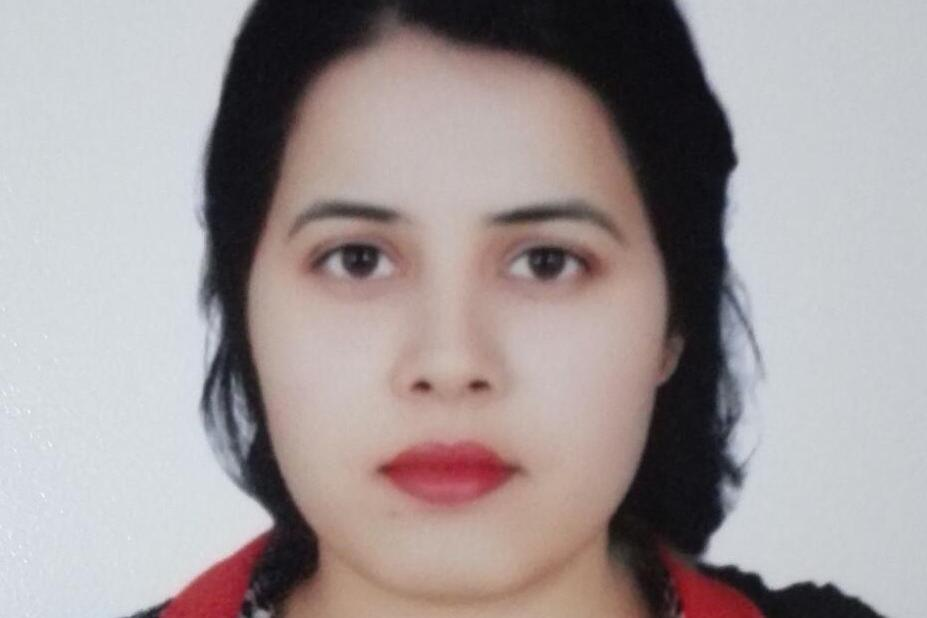Event date:
Oct 22 2021 2:30 pm

## A framework where conventional computational fluid dynamics (CFD) meets machine learning

Supervisor
Dr. Zahra Lakdawala
Student
Kinza Mumtaz
Venue
Zoom Meetings (Online)
Event
PhD Research Seminar

Abstract:

Computational fluid dynamics(CFD) is one of the most well known methods for solving fluid flow problems. However, they are expensive in terms of costs, time and energy. We are exploring how techniques from deep learning can be applied for the models that describe fluid flow behavior, in a way that tackles the fundamental limitations of current CFD techniques. In our talk, we will introduce an idea of ,  that describes a physics-informed neural network framework. Our focus is to understand how to embed the physical laws (in the form of complex PDES) in the training of neural networks that are then used to solve supervised learning tasks. For a deeper understanding of the process, we will cover the concept of numerical Gaussian process, which is constructed as a Gaussian process with covariance function resulting from discretization of spatio-temporal partial differential equations. We will use Burger’s equation to illustrate the proof of concept.

Publications:

 M. Raissi and G. E. Karniadakis. Hidden physics models: machine learning of nonlinear partial differential equations. J. Comput. Phys., 357, 2018, 125-141.

 M. Raissi, P.  Perdikaris and G. E. Karniadakis. Physics-Informed Neural Networks: A Deep Learning Framework for Solving Forward and Inverse Problems Involving Nonlinear Partial Differential Equations. J. Comput. Phys.,2019,  686-707.

ZOOM Information:

Meeting ID:                 992 7390 0408

Passcode:                     868444